# Interactive Demonstrations for Statistics Education on the World Wide Web

R. Webster West and R. Todd Ogden
University of South Carolina

Journal of Statistics Education v.6, n.3 (1998)

Copyright (c) 1998 by R. Webster West and R. Todd Ogden, all rights reserved. This text may be freely shared among individuals, but it may not be republished in any medium without express written consent from the authors and advance notification of the editor.

# Power of a Hypothesis Test Applet

This applet illustrates the fundamental principles of statistical hypothesis testing through the simplest example: the test for the mean of a single normal population, variance known (the Z test).

The basic set-up of the test is this: using only n independent observations X1, X2,..., Xn from a normal distribution with unknown mean (but known variance), the task is to decide whether or not to reject a null hypothesis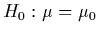in favor of some alternative hypothesis. In most applications, there are only three alternative hypotheses of interest:

1.2.3.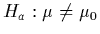These hypotheses are, respectively, "upper-tailed," "lower-tailed," and "two-tailed." The testing framework consists of computing a test statistic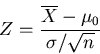,

and then rejecting the null hypothesis if the appropriate condition is satisfied. In the order of the alternative hypotheses above, the null hypothesis is rejected if

1.,
2.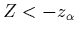,
3.,
where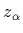represents the upper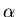critical point of the standard normal distribution.

This hypothesis testing procedure is set up to give the null hypothesis the "benefit of the doubt;" that is, to not reject the null hypothesis unless there is strong evidence to support the alternative. If H0 is true, the above test statistic follows a standard normal distribution, so the probability of erroneously rejecting H0 is just. If Ha is true, however, the test statistic Z does not follow a standard normal distribution -- it follows a normal distribution with a different mean, and thus, the probability of (correctly) rejecting the null hypothesis is larger than. This probability is knows as the power of the test, and it depends on the true value of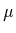. (Clearly, a test would have more power for an extreme value ofthan for athat is very close to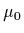.)

To use this applet, you must specify the hypothesized mean, the true mean, and the value of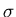, and select the appropriate alternative hypothesis. Clicking on the Show it! button will give a plot. The black curve represents the distribution of the test statistic when the null hypothesis is true. The portion shaded in red represents the probability of falling beyond the cut-off point(s) when the null hypothesis is true (the Type I error rate, or). The blue curve represents the distribution of the test statistic under the particular value ofyou selected. The blue shaded area represents the power of the test for that particular value of. Note that the region shaded both blue and red appears purple.